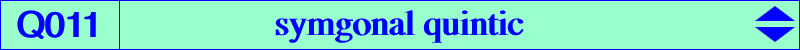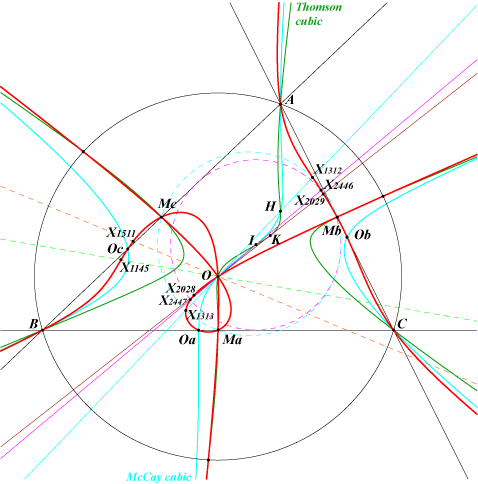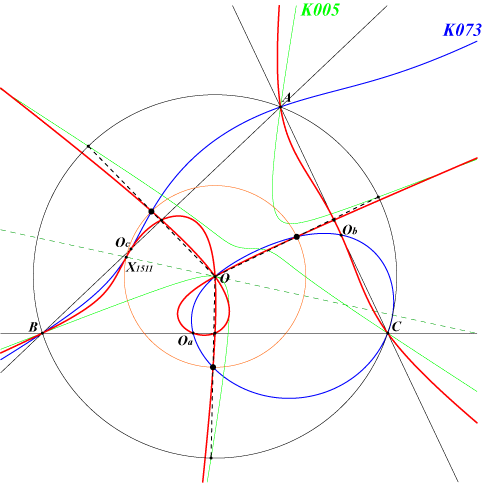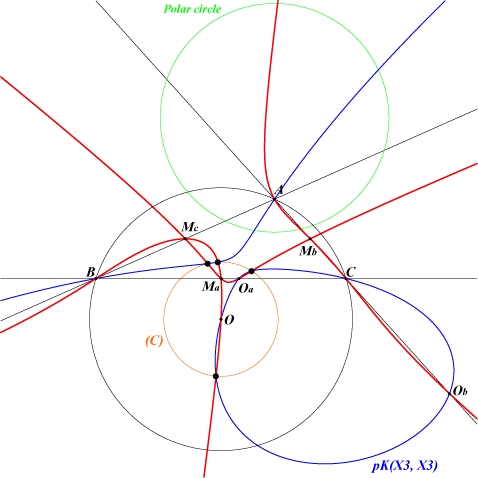too complicated to be written here. Click on the link to download a text file.X(3) triple point X(1145), X(1312), X(1313), X(1511), X(2028), X(2029), X(2446), X(2447), X(5976), details below. extraversions of X(1145), X(2446), X(2447) Ma, Mb, Mc : midpoints of ABC (nodes) Oa, Ob, Oc : vertices of the cevian triangle of O points at infinity of the McCay cubic common points of the circumcircle and the Thomson cubic i.e. vertices of the Thomson triangleQ011 is the locus of point P such that P, its symgonal Q and O are collinear (together with the line at infinity, although the symgonal of a point at infinity is itself). Q011 is a circular quintic with singular focus O which is a triple point on the curve. It has three real asymptotes which are those of the McCay cubic. The tangents at A, B, C concur at X(25). Bui Quang Tuan has found that Q011 is the locus of the intersection Q of the line OP and the Simson line S(P) of P when P traverses the circumcircle (see Hyacinthos #14759). Note that, if the Simson line is replaced by the Steiner line, the locus is K028, the Musselman (third) cubic. Thus, for P = u:v:w on the circumcircle, this point Q is : b^2c^2u^4(c^2v^2–b^2w^2)(c^2SC v–b^2SB w) : : . Now, if P' is the antipode of P on the circumcircle, the line L(P) = OPP' and the Simson line S(P') of P' meet at Q' obviously on Q011. S(P) and S(P') meet at X on the nine point circle and the circle C(P) with diameter QQ' is tangent at X to the nine point circle. The center Ω of C(P) lies on K258, the complement of K028. The barycentric product QxQ' of Q and Q' lies on the Steiner inscribed ellipse and the points X(115), X, QxQ' are collinear. We obtain the following special cases :L(P) C(P) X Ω points Q, Q' on Q011 Euler line nine points circle X(125) X(5) X(1312), X(1313) Brocard axis Moses circle X(115) X(39) X(2028), X(2029) line OI incircle X(11) X(1) X(2446), X(2447) lines O-excenters excircles extraversions of X(11) excenters extraversions of X(2446), X(2447) line O X(8) X(1145) and X(1145)' line O X(74) X(1511) and X(1511)'Notes : X(1145)' = (b - c)^2 (b + c - 2a) / [(b + c)(a + b - c)(a - b + c) - 2abc], SEARCH = 4.0046105891, on the lines X(3)X(8), X(11)X(513). X(1511)' = a^2 (b^2 - c^2)^2 (2SA + b c)*(2SA - b c) / [a^2 (2SA - a^2) + (b^2 - c^2)^2)], SEARCH =3.9490387472, on the lines X(3)X(74), X(125)X(523).Intersection of Q011 and pK(Ω, O) Let pK = pK(Ω, O) be a pivotal isocubic with pole Ω, pivot O. pK and Q011 meet at 15 points namely A, B, C, O (triple), Oa, Ob, Oc and six other points depending of Ω. When Ω lies on the Brocard axis OK, these six points lie on a same circle (C) with center O, although these points might not be all real nor distinct. See CL021 for pivotal cubics with pivot O and specially those with pivot on OK. If k is a positive real number and kR the radius of the circle (R is the circumradius), the coordinates of the corresponding pole Ω are a^2(cos^2 A – k^2) : : .Special cases 1. When k = 0, Ω is X(577), the barycentric square of O. The cubic decomposes into the cevian lines of O and the circle (C) reduces to O itself. 2. When k = 1, Ω is X(32), the third power point, and pK is K172. (C) is the circumcircle itself and the six points are A, B, C again and the points where K172 and the Thomson cubic meet the circumcircle. 3. When k is infinite, Ω is the Lemoine point X(6) and pK is the McCay cubic. (C) degenerates into the line at infinity counted twice. 4. When k = 1/2, Ω is X(50) and pK is K073 or Ki which is a circular cubic passing through X(1511) also on Q011. It follows that Q011 and K073 must have three other common points on the circle (C) with radius R/2. These three points are the midpoints of O and the three common points (apart A, B, C) of the circumcircle and the Napoleon cubic K005. See the figure opposite.5. When k^2 = – cosA cosB cosC, Ω = O and the cubic is pK(X3, X3). (C) is real when ABC is obtuse angle and its radius is half that of the polar circle. The six common points are not necessarily all real. The figure opposite shows four real points.